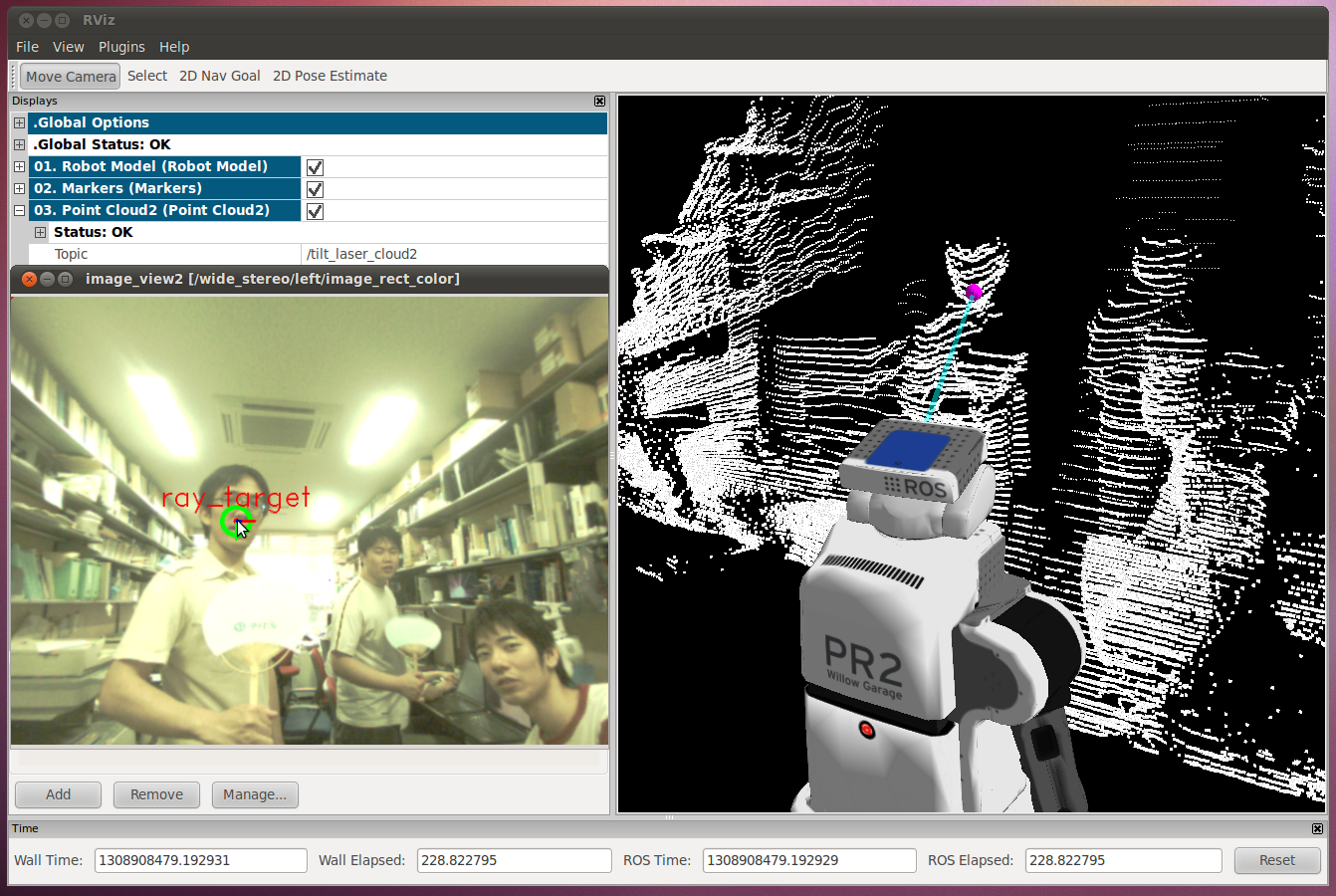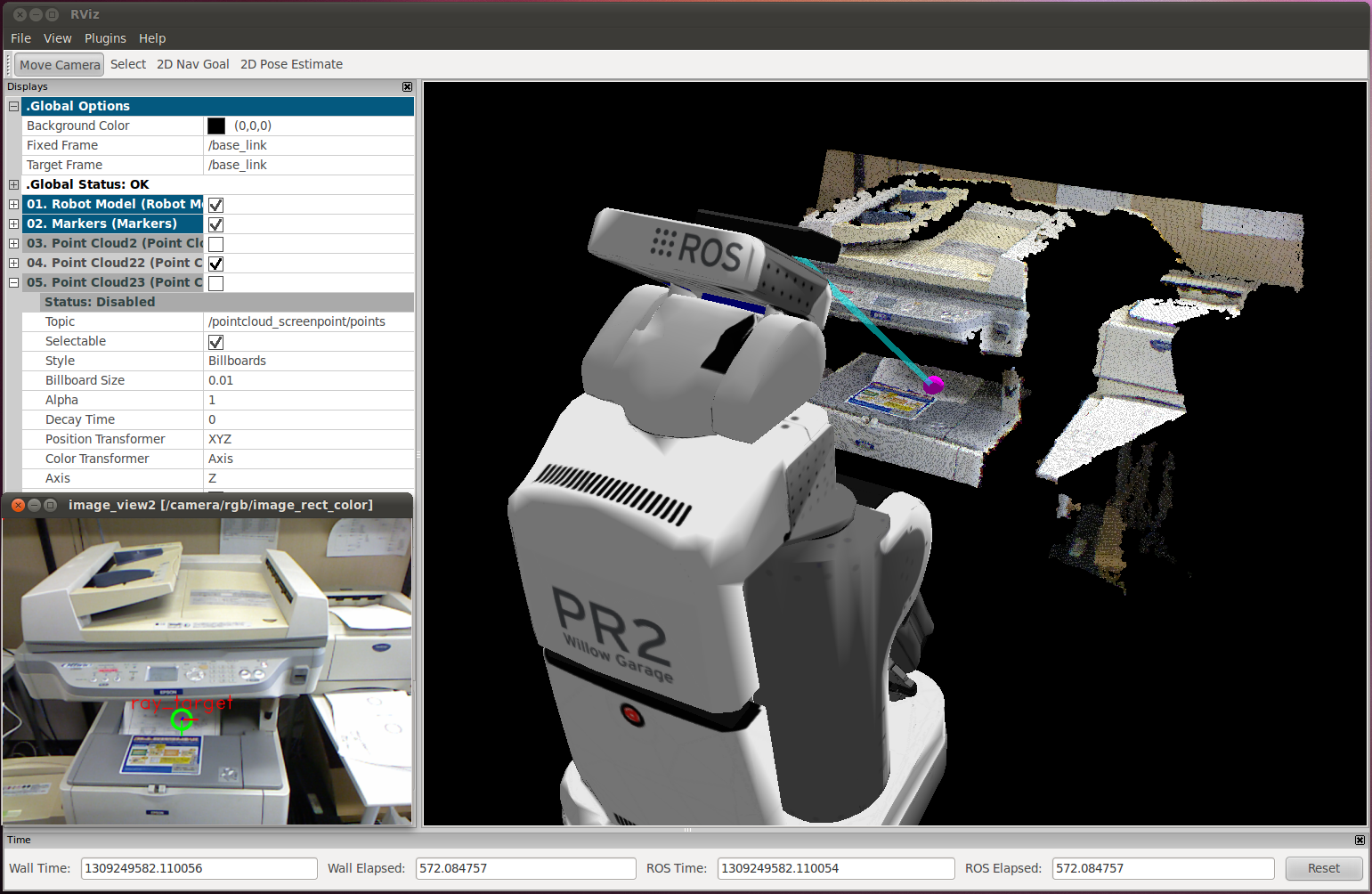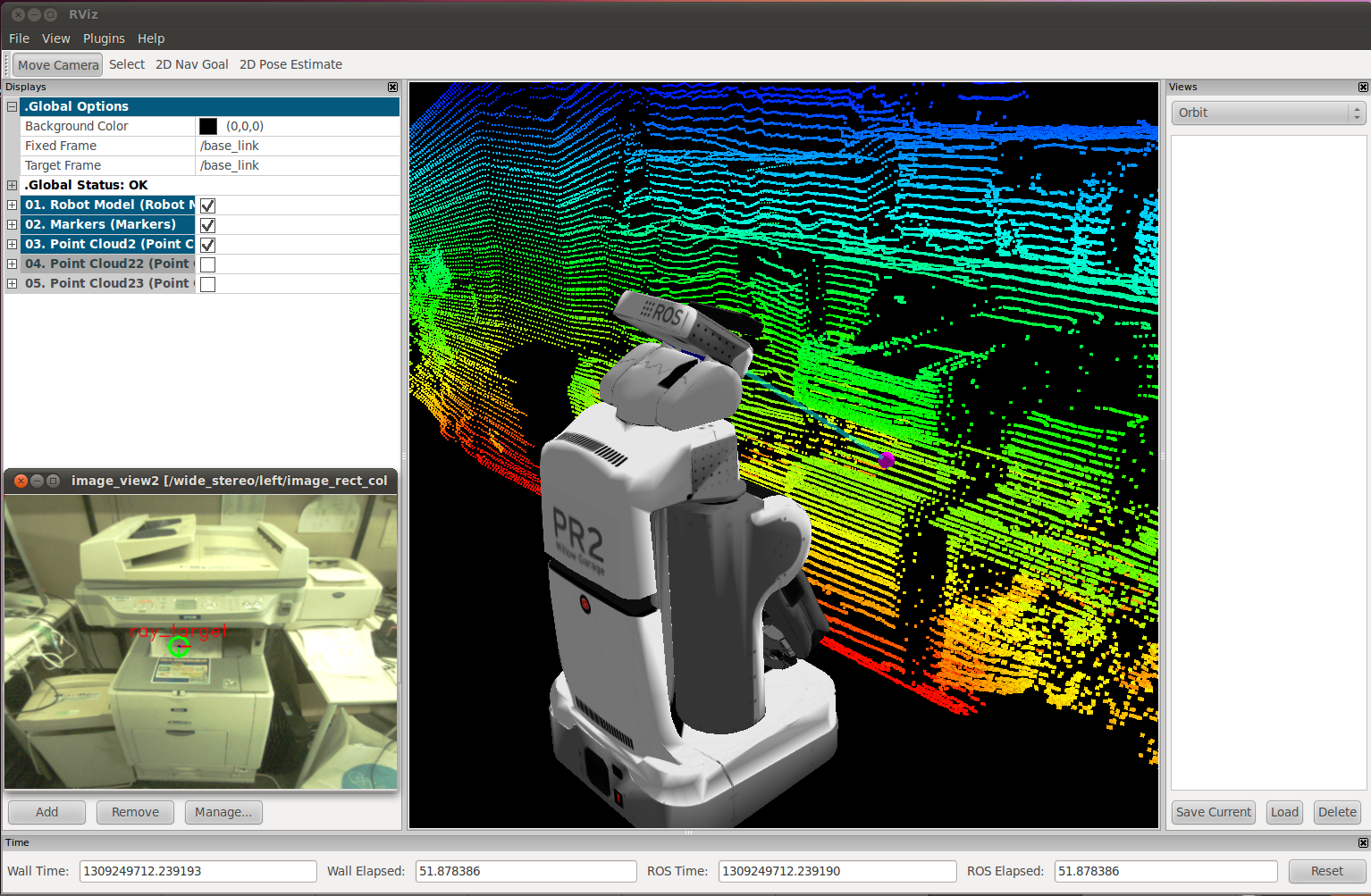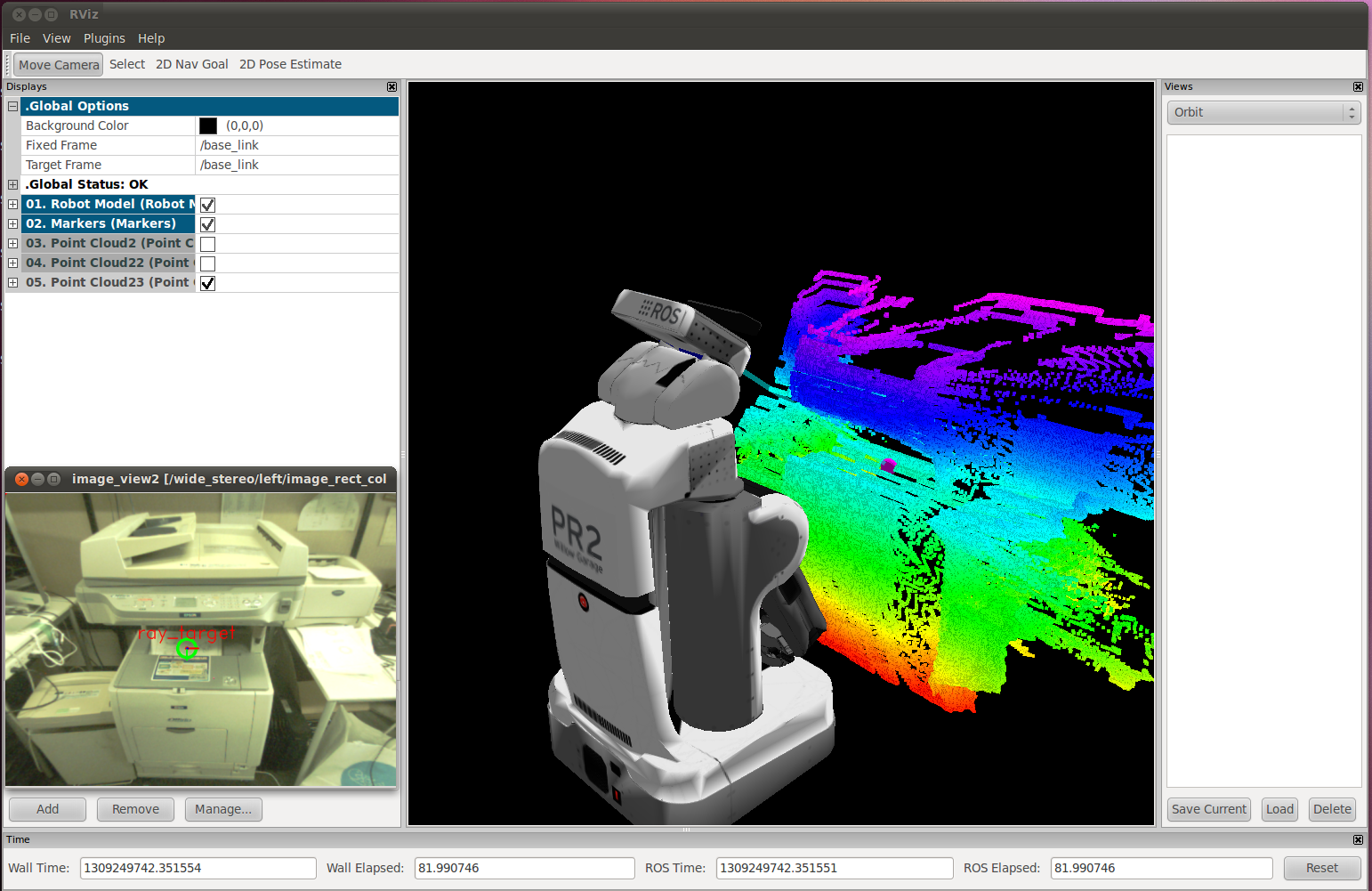# PointcloudScreenpoint¶

## What is this¶Use pointcloud from kinectUse pointcloud from laserThis is a nodelet to convert (u, v) coordinate on a image to 3-D point. It retrieves 3-D environment as pointcloud.

pointcloud_screenpoint_sample.launch is a sample launch file.

## Note¶

This class inherits jsk_topic_tools::ConnectionBasedNodelet, which can start subscribing topics if published topics are subscribed by the other node.

## Subscribing Topics¶

• ~points (sensor_msgs/PointCloud2):

Pointcloud source to estimate 3D points that the user wantedt to specify on a 2D screen

• ~point (geometry_msgs/PointStamped):

Input point to represent (u, v) image coordinate. Only x and y fileds are used and the header frame_id is ignored. If ~synchronization parameter is set True, ~points and ~point are synchronized.

• ~rect (geometry_msgs/PolygonStamped):

Input rectangular region on image local coordinates. Only x and y fields are used and the header frame_id is ignored. And the region should be rectangular. If ~synchronization parameter is set True, ~points and ~rect are synchronized.

• ~poly (geometry_msgs/PolygonStamped):

Input polygonal region in image local coordinates. If ~synchronization parameter is set True, ~points and ~poly are synchronized.

• ~point_array (sensor_msgs/PointCloud2):

Input points to represent series of (u, v) image coordinate. Only x and y fields are used and the header frame_id is ignored. If ~synchronization parameter is set True, ~points and ~point_array are synchronized.

## Publishing Topics¶

• ~output_point (geometry_msgs/PointStamped):

The topic to be used to publish one point as a result of screenpoint.

• ~output (sensor_msgs/PointCloud):

The topic to be used to publish series of points as a result of screenpoint.

• ~output_polygon (geometry_msgs/PolygonStamped):

Projected points of ~rect or ~poly.

• ~screen_to_point (jsk_pcl_ros::TransformScreenpoint):

ROS Service interface to convert (u, v) image coordinate into 3-D point.

The definition of jsk_pcl_ros::TransformScreenpoint is:

# screen point
float32 x
float32 y
---
# position in actual world
geometry_msgs/Point point
geometry_msgs/Vector3 vector


With int this service, the latest pointcloud acquired by ~points is used to convert (u, v) into 3-D point.

## Parameters¶

• ~synchronization (Boolean, default: False):

If this parameter is set to True, the timestamps of 3-D pointcloud and the target point/rectangle/point array are synchronized.

• ~approximate_sync (Boolean, default: False):

If this parameter is set to True, approximate synchronization is enabled. This parameter is valid only when ~synchronization is True.

• ~queue_size (Integer, default: 1):

Queue length of subscribing topics.

• ~crop_size (Integer, default: 10):

The size of approximate region if ~points pointcloud has nan holes.

• ~search_size (Integer, default: 16):

Size to search normal of point cloud.

• ~timeout (Double, default: 3.0`):

Timeout to wait for point cloud.